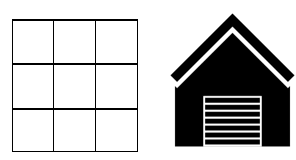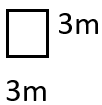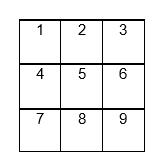# Math Worksheets Land

Math Worksheets For All Ages

# Math Worksheets Land

Math Worksheets For All Ages

# Measure Area By Counting Units Worksheets

When you begin to do your first construction project in your home you will need to revisit this skill. That is because most building materials are sold in units of square area. There are many different techniques that you can use to determine this value. In this section we will explore a very basic way to go about this. It can also be used to breakdown larger and more complex irregular geometric figures. These worksheets help students learn to measure total area by finding the sum of all the area of separate figures. You will find this a good starting point for most students.

### Aligned Standard: Grade 3 Measurement - 3.MD.7

• Using Units Step-by-step Lesson- Use one repeated unit to determine the area of the entire structure. This lends itself to all forms of geometry.
• Guided Lesson - You first find the total area of a figure with repeated units and then you find the area of one unit of a large figure.
• Guided Lesson Explanation - We break it all down for you. This is one that you will want to print out.
• Practice Worksheet - Half of the worksheet involves finding total area of a figure. The other half is finding one unit of the figure.
• Matching Worksheet - Match the areas to the rectangles they discuss.
• Answer Keys - These are for all the unlocked materials above.

### Homework Sheets

I do this every time I need to tile my bathroom.

• Homework 1- Squares are regular quadrilateral. Since the two adjacent sides have equal length, we can multiply both the sides to determine the area of one square.
• Homework 2- Look at the figure below and find out the adjacent sides of each square.
• Homework 3-The area of the square they want us to draw is 32 cm.

### Practice Worksheets

If you ever work on your own home, this skill will be so useful to have.

• Practice 1- What is the area of the figure above?
• Practice 2- Find out the adjacent sides of each square.
• Practice 3- Draw the shape of a figure composed of these squares that has an area of 72 cm.

### Math Skill Quizzes

There is one question on every quiz that takes longer than all the other questions combined.

• Quiz 1- What is the area of the figure below?
• Quiz 2- Draw the shape of a figure composed of these squares that has an area of 294 cm.
• Quiz 3- Find out the adjacent sides of each square.

### Quick ways to Measure Area by Counting Rows and Columns

Calculating area is one of the most important concepts in mathematics. It is one of those concepts that has its uses in your daily life. For example, when you go out to purchase a carpet for your living room, you will have to provide the shopkeeper with the approximate area of your living room so that they can determine how much carpet you will need to purchase. Moreover, if you go to buy a table cover, you need to know the total area of the table for a cover so that will perfectly fit. If you are not confident with these measures you will simply waste money and have poorly fitted carpet and table covers.

Kids might find it difficult to calculate area of the two-dimensional shapes. There are a few techniques which make area calculation very easy for kids and one technique is by counting the rows and columns. This technique is useful for regular polygons including squares and rectangles. They can also be applied to complex shapes that can be broken down into these geometric shapes.

You can start by dividing the shape into square centimeters, millimeters, or meters, as per the given the dimensions and measurement units you are using. When you successfully divide your shape into rows and columns, all you have to do is count the rows and write it down. Then you must count the number of columns. To get the final answer you just multiply the number of rows with the columns to get the final answer. Let’s see how this applies to an everyday problem.

### Example Problem:

You have decided that the floor of your garage could use some color to spruce it up. You are determining the square area of your garage floor to determine how much paint you will need. You will see a diagram of the garage floor below.If each square is equal to the following:What is the area of the garage floor?

### Solution:

I would begin by determine the area of one square. The area of a square is side2. One side, according to the diagram is equal to 3 meters. This means the area of one square would be 32 = 9 meters. The next thing we will need to do is determine how many of these squares makes up the entire garage floor. We can do this by simply counting. You can see this in the diagram below.This means that there are 9 total squares that each have the area of 9 meters. The total area would therefore by 9 x 9 = 81 meters2.

Unlock all the answers, worksheets, homework, tests and more!
Save Tons of Time! Make My Life Easier Now

## Thanks and Don't Forget To Tell Your Friends!

I would appreciate everyone letting me know if you find any errors. I'm getting a little older these days and my eyes are going. Please contact me, to let me know. I'll fix it ASAP.